# Indirect MRAC Control of Mass-Spring-Damper System

This example shows how to use an indirect model reference adaptive control (MRAC) system in Simulink for model parameter estimation of a second-order mass-spring-damper system. The properties of the mass-spring-damper system are unknown. The indirect MRAC controller estimates the plant parameters and implements an inversion-based controller to track a reference model.

### Mass-Spring-Damper Model

The mass-spring-damper system consists of two carts of mass ${\mathit{m}}_{1}$ and ${\mathit{m}}_{2}$, connected to each other and ground through springs with stiffness coefficients ${\mathit{c}}_{0}$ and ${\mathit{c}}_{1}$ and dampers with damping coefficient $d$.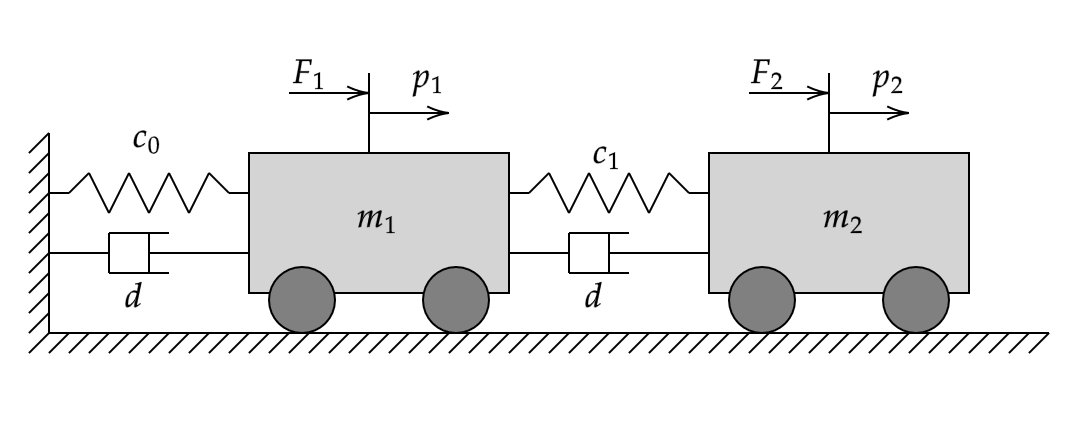The unknown dynamical system defining the spring-mass-damper system can be written as follows.

`$\stackrel{˙}{\text{\hspace{0.17em}\hspace{0.17em}}\mathit{x}\left(\mathit{t}\right)}=\mathit{Ax}\left(\mathit{t}\right)+\mathit{Bu}\left(\mathit{t}\right)$`

`$\mathit{A}=\left[\begin{array}{cccc}0& 1& 0& 0\\ -\frac{\left({\mathit{c}}_{0}+{\mathit{c}}_{1}\right)}{{\mathit{m}}_{1}}& -\frac{2\mathit{d}}{{\mathit{m}}_{1}}& \frac{{\mathit{c}}_{1}}{{\mathit{m}}_{1}}& \frac{\mathit{d}}{{\mathit{m}}_{1}}\\ 0& 0& 0& 1\\ \frac{{\mathit{c}}_{1}}{{\mathit{m}}_{2}}& \frac{\mathit{d}}{{\mathit{m}}_{2}}& -\frac{{\mathit{c}}_{2}}{{\mathit{m}}_{2}}& -\frac{2\mathit{d}}{{\mathit{m}}_{2}}\end{array}\right],\text{\hspace{0.17em}}\mathit{B}=\left[\begin{array}{cc}0& 0\\ \frac{1}{{\mathit{m}}_{1}}& 0\\ 0& 0\\ 0& \frac{1}{{\mathit{m}}_{2}}\end{array}\right]$`

Here:

• $\mathit{x}=\left[{\mathit{p}}_{1},\stackrel{˙}{{\mathit{p}}_{1}},{\mathit{p}}_{2},\stackrel{˙}{{\mathit{p}}_{2}}\right]$ is the system state vector.

• ${\mathit{p}}_{1}$ and ${\mathit{p}}_{2}$ are the positions of the masses.

• $\mathit{A}$ and $\mathit{B}$ are the parameters of the unknown system.

Given this unknown nonlinear system, the goal is to design a controller that enables the tracking of the following reference.

`$\stackrel{˙}{{\mathit{x}}_{\mathit{m}}}\left(\mathit{t}\right)={\mathit{A}}_{\mathit{m}}{\mathit{x}}_{\mathit{m}}\left(\mathit{t}\right)+{\mathit{B}}_{\mathit{m}}\mathit{r}\left(\mathit{t}\right)$`

`${\mathit{A}}_{\mathit{m}}=\left[\begin{array}{cccc}0& 1& 0& 0\\ -25& -10& 0& 0\\ 0& 0& 0& 1\\ 0& 0& -25& -10\end{array}\right],{\mathit{B}}_{\mathit{m}}=\left[\begin{array}{cc}0& 0\\ 25& 0\\ 0& 0\\ 0& 25\end{array}\right]$`

Here:

• ${\mathit{x}}_{\mathit{m}}$ contains the reference model states.

• ${\mathit{A}}_{\mathit{m}}$ and ${\mathit{B}}_{\mathit{m}}$ are the parameters of the reference system.

• $\mathit{r}\left(\mathit{t}\right)$ is the reference signal provided by the user.

### Reference-Tracking Controller

The indirect MRAC controller uses an estimator model to compute $\underset{}{\overset{ˆ}{A}}$ and $\underset{}{\overset{ˆ}{B}}$, which are estimates of the unknown system parameters $\mathit{A}$ and $\mathit{B}$, respectively.

`$\underset{}{\overset{˙}{\underset{}{\overset{ˆ}{x}}}}=\underset{}{\overset{ˆ}{A}}x\left(t\right)+\underset{}{\overset{ˆ}{B}}u\left(t\right)$`

The compute the control action $\mathit{u}\left(\mathit{t}\right)$, the controller uses the feedforward gain ${\mathit{k}}_{\mathit{r}}$ and feedback gain ${\mathit{k}}_{\mathit{x}}$.

`$u\left(t\right)=-{k}_{x}x\left(t\right)+{k}_{r}r\left(t\right)$`

The controller gains are derived from the reference model parameters (${\mathit{a}}_{\mathit{m}}$ and ${\mathit{b}}_{\mathit{m}}$) and estimated observer parameters ($\underset{}{\overset{ˆ}{a}}$ and $\underset{}{\overset{ˆ}{b}}$).

`$\begin{array}{l}{k}_{r}=\frac{{B}_{m}}{\underset{}{\overset{ˆ}{B}}}\\ {k}_{x}=\frac{1}{\underset{}{\overset{ˆ}{B}}}\left({A}_{m}-\underset{}{\overset{ˆ}{A}}\right)\end{array}$`

### Configure Controller

Specify the true stiffness coefficients, damping coefficient, and masses for the mass-spring-damper system.

```% Stiffness c0 = 1; c1 = 1; % Damping d = 1; % Mass m1 = 5; m2 = 1;```

Define the actual system dynamics using these system parameters..

```A = [0 1 0 0;-(c0+c1)/m1 -2*d/m1 c1/m1 d/m1; 0 0 0 1;c1/m2 d/m2 -c1/m2 -2*d/m2]```
```A = 4×4 0 1.0000 0 0 -0.4000 -0.4000 0.2000 0.2000 0 0 0 1.0000 1.0000 1.0000 -1.0000 -2.0000 ```
`B = [0 0;1/m1 0;0 0;0 1/m2]`
```B = 4×2 0 0 0.2000 0 0 0 0 1.0000 ```

This true model is unknown to the indirect MRAC controller. Instead, the controller uses an estimator model to estimate the unknown plant dynamics. During operation, the controller can adapt the parameters of this model to improve its estimate of the unknown system parameters.

```Ahat = [0 1 0 0;0 0 0 0;0 0 0 1;0 0 0 0]; Bhat = [0 0.1;0.1 0;0 0;0.1 0.1];```

The goal of the controller is to track the performance of the reference model. Specify the parameters of the reference model.

```Am = [0 1 0 0;-25 -10 0 0;0 0 0 1;0 0 -25 -10]; Bm = [0 0;25 0;0 0;0 25];```

Specify the initial condition of the plant.

`x_0 = 0;`

Specify the learning rates for updating the estimator model parameters.

```gamma_a = 0.1; % Ahat learning rate gamma_b = 0.1; % Bhat learning rate```

### Simulate Controller

```mdl = 'mracMassSpringDamper'; open_system(mdl)```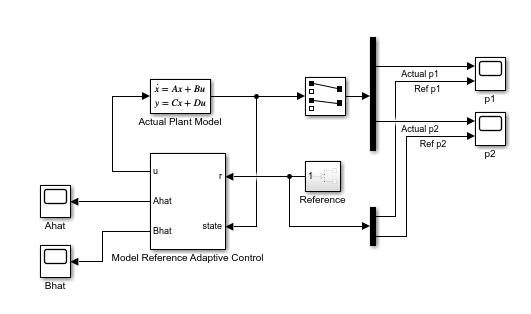In this model:

• The Actual Plant Model block implements the nominal model of the mass-spring-damper system.

• The Reference block generates reference signals for both masses.

• The Model Reference Adaptive Control block outputs the control action u, which it derives from the using the estimator model.

While an MRAC controller can also estimate unknown disturbances in the controlled system, for this example there are no such disturbances. Instead, the goal of the controller is simply to estimate the parameters of the unknown plant model. For an example that estimates unknown disturbances using a direct MRAC controller, see Model Reference Adaptive Control of Aircraft Undergoing Wing Rock.

Simulate the model.

```Tf = 100; sim(mdl);```

View the actual plant states, that is the positions of the masses, along with the corresponding reference signals. The controller is able to make the actual plant states track the reference signals.

`open_system(mdl + "/p1")`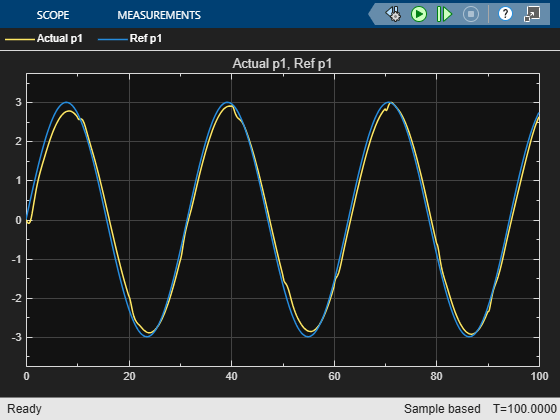`open_system(mdl + "/p2")`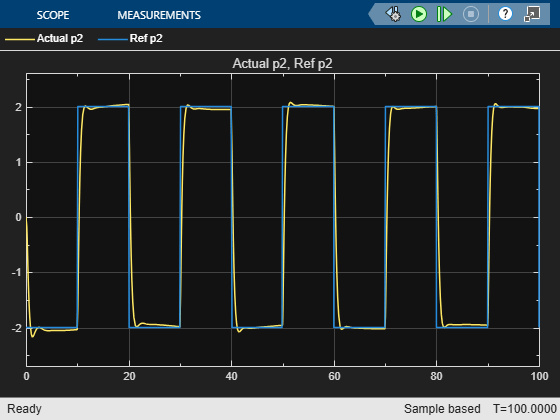The Model Reference Adaptive Controller block is configured to output the parameters $\underset{}{\overset{ˆ}{A}}$ and $\underset{}{\overset{ˆ}{B}}$ of the estimator model using the `Ahat` and `Bhat` output ports, respectively. Plot the parameters.

`open_system(mdl + "/Ahat")`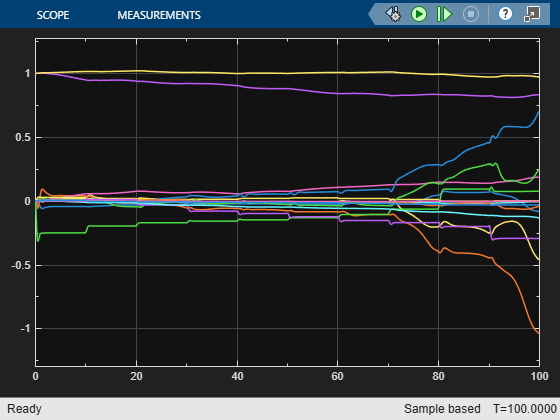`open_system(mdl + "/Bhat")`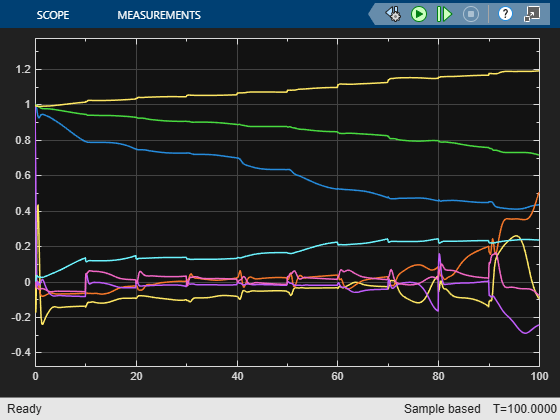Over time the controller adapts the values of the estimator parameters. However, the estimated parameters do not converge to the true parameters due to a lack of persistency of excitation in the reference signals. Despite the fact that the model parameters do not converge, the controller still converges to the reference behavior.